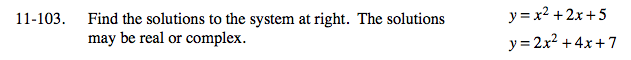Home > A2C > Chapter 11 > Lesson 11.2.5 > Problem11-103

11-103.

Find the solutions to the system below. The solutions may be real or complex. Homework Help ✎ y = x2 + 2x + 5
y = 2x2 + 4x + 7−2(y = x2 + 2x + 5) → −2y = −2x2 − 4x − 10
y = 2x2 + 4x + 7

y = −3
y = 3

Use substitution to find x.

(3) = x2 + 2x + 5

0 = x2 + 2x + 2

Use the quadratic formula.

(−1 + i, 3) and (−1 − i, 3)